# FunctionsIn this topic, we will look at

• Composite and Inverse Functions
• Quadratic Functions and the Discriminant
• Rational Functions
• Transforming Functions
• The Factor and Remainder Theorem
• Sums and Products of Polynomial Equations

•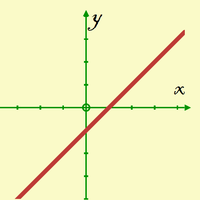#### Equation of a Straight Line

You will probably recognise the most commonly used form of the equation of a straight line: y = mx + c where m represents the gradient and c represents the y-intercept. It is important not to overlook this topic, as it comes up a lot in work on Functions,

•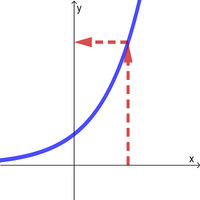#### Functions - The Basics

In this page, we will look at the different types of relations including functions. The first step to gaining a good understanding of functions is to think about inputs and outputs. Once that is clear, you should be able to deal with domain...

•On this page we will look at quadratic expressions and how the different factorised forms link to the shape of the graph. We will also get an understanding about how the discriminant affects not only the number of roots of a quadratic equation, but also ho

•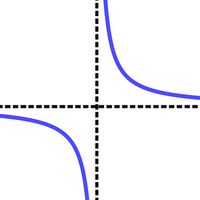#### Rational Functions SL

On this page, we will look at the properties of the reciprocal function and the rational function. You may be required to draw a sketch of these functions. In these cases, it is important to know how to find the vertical asymptote, the horizontal...

•#### Rational Functions HL

On this page, we will look at the properties of the reciprocal function and rational functions. You may be required to draw a sketch of these functions. In these cases, it is important to know how to find the vertical asymptote, the horizontal...

•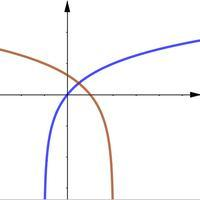#### Transforming Functions SL

For the topic of transforming functions, we need to understand the effect of translating, reflecting and stretching has on functions. You may be asked to describe the transformations, sketch graphs or find the coordinates of points that have been transform

•#### Transforming Functions HL

For the topic of transforming functions, we need to understand the effect of translating, reflecting and stretching has on functions. You may be asked to describe the transformations, sketch graphs or find the coordinates of points that have been transform

•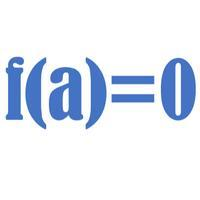#### Factor and Remainder Theorem

The factor theorem is a special case of the remainder theorem, in that (x-a) is a factor if and only if P(a)=0

•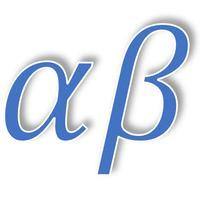#### Sums and Products of Roots

On this page, we learn all about roots of polynomial equations and how to use the formulae for the sum of the roots and the product of the roots.